### The Form of the Finite Mixture Model

Suppose that you observe realizations of a random variable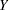, the distribution of which depends on an unobservable random variable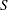that has a discrete distribution.can occupy one ofstates, the number of which might be unknown but is at least known to be finite. Sinceis not observable, it is frequently referred to as a latent variable.

Let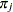denote the probability thattakes on state. Conditional on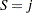, the distribution of the responseis assumed to be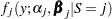. In other words, each distinct stateof the random variableleads to a particular distributional form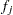and set of parameters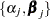for.

Let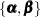denote the collection of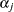and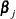parameters across all=to. The marginal distribution ofis obtained by summing the joint distribution ofandover the states in the support of: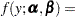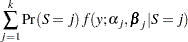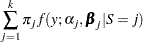This is a mixture of distributions, and theare called the mixture (or prior) probabilities. Because the number of statesof the latent variableis finite, the entire model is termed a finite mixture (of distributions) model.

The finite mixture model can be expressed in a more general form by representing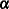andin terms of regressor variables and parameters with optional additional scale parameters for. The section Notation for the Finite Mixture Model develops this in detail.